# Math in Focus Grade 8 Chapter 11 Review Test Answer Key

This handy Math in Focus Grade 8 Workbook Answer Key Chapter 11 Review Test detailed solutions for the textbook questions.

## Math in Focus Grade 8 Course 3 B Chapter 11 Review Test Answer Key

Concepts and Skills

State whether each event is a simple or compound event.

Question 1.
Drawing 2 yellow marbles ¡n a row from a bag of yellow and green marbles.
Drawing 2 yellow marbles in a row from a bag of yellow and green marbles are compound events. Because the number of marbles is 3 so, the result is the number of outcomes.

Question 2.
Drawing 1 red pebble and 1 yellow pebble in a row from a bag of red and yellow pebbles.
Drawing 1 red pebble and 1 yellow pebble in a row from a bag of red and yellow pebbles is compound. Because the number of pebbles is 2 so, the result is the number of outcomes

Question 3.
Tossing a coin once.
Tossing a coin once is a simple event because it has a result of one outcome.

Draw the possibility diagram and state the number of possible outcomes for each compound event.

Question 4.
From three cards labeled A, B, and C, draw two cards, one at a time with replacement.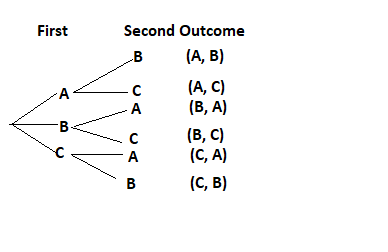Question 5.
From a pencil case with 1 red pen, 1 green pen, and 1 blue pen, select two pens, one at a time without replacement.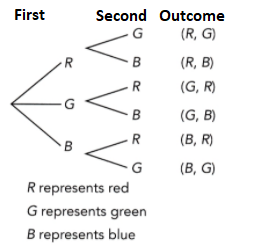Question 6.
Toss a fair four-sided number die, labeled 1 to 4, and a coin.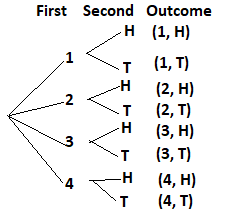Draw the tree diagram for each compound event.

Question 7.
Spinning a spinner divided into 4 equal areas labeled 1 to 4, and tossing a coin.Question 8.
Picking two green apples randomly from a basket of red and green apples.
The probability of picking red and green apples is 1/2.

State whether each compound event consists of independent events or dependent events.

Question 9.
From a pencil case, two-color pencils are randomly drawn, one at a time without replacement.

Question 10.
From two classes of 30 students, one student ¡s selected randomly from each class for a survey.

Problem Solving

Question 11.
There are two tables in a room. There are 2 history textbooks and 1 math textbook on the first table. There are 1 history workbook and 1 math workbook on the second table. Use a possibility diagram to find the probability of randomly selecting a history textbook from the first table and a math workbook from the second table.
Given,
There are two tables in a room. There are 2 history textbooks and 1 math textbook on the first table.
1 + 2 = 3
The probability of randomly selecting a history textbook from the first table and a math workbook from the second table is 1/3.

Question 12.
A fair four-sided number die is marked 1, 2, 2, and 3. A spinner equally divided into 3 sectors is marked 3, 4, and 7. Jamie tosses the number die and spins the spinner.
a) Use a possibility diagram to find the probability that the sum of the two resulting numbers is greater than 5.
Note that there are 4 possible outcomes in rolling a die which is 1, 2, 2, and 3, and 3 outcomes for the spinner which are 3, 4 and 7. Add these possible resuLts, then mark the answers that are bigger the 5.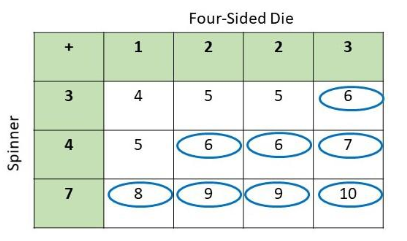Observe that there are 12 possible outcomes and 8 are greater than 5 then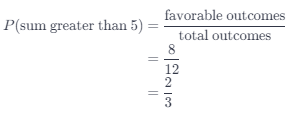Therefore, the probability of obtaining a number that is larger than 5 is $$\frac{2}{3}$$.

b) Use a possibility diagram to find the probability that the product of the two resulting numbers is odd.
Notice that there are 4 possible outcomes in rolling a die which is 1, 2, 2. and 3, and 3 outcomes for the spinner which are 3, 4 and 7. Multiply these possible results, then mark the answers that odd numbers.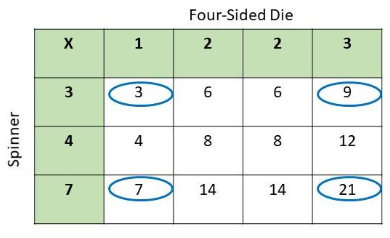Observe that there are 12 possibLe outcomes and 4 are odd numbers then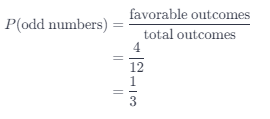Therefore, the probability of obtaining an odd number is $$\frac{1}{3}$$.

Question 13.
A juggler is giving a performance by juggling a red ball, a yellow ball, and a green ball. All 3 balls have equal chance of dropping. If one ball drops, the juggler will stop and pick up the ball and resume juggling. If another ball drops again, the juggler will stop the performance.
a) Draw a tree diagram to represent the possible outcomes and the corresponding probabilities.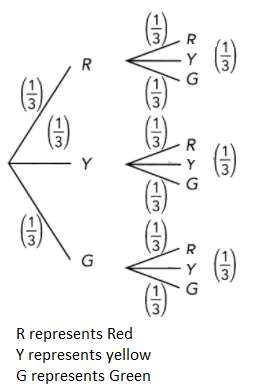b) Find the probability of dropping the same colored ball twice.

c) Find the probability of dropping one green and one yellow ball.
P(G)P(R) = 1/3 × 1/3= 1/9

Question 14.
In a marathon, there is a half-marathon and a full-marathon. There are 60 students who participated in the half-marathon and 80 participated in the full-marathon. Half of the students in the half-marathon warm up before the run, while three-quarters of the students in the full-marathon warm up. Assume that warming up and not warming up are mutually exclusive and complementary.
a) Draw a tree diagram to represent the possible outcomes and the corresponding probabilities.
Since, there are 2 types of marathon, then start the tree diagram with 2 branches. Now, at the end of these branches put the possible outcomes: H for half-marathon, and F for full marathon. Note that the probability of full marathon is $$\frac{80}{140}$$ and the probability of half-marathon is $$\frac{60}{140}$$. Next, there are a group who warms up and who does not, then make another 2 branches. At the end of these branches put the possible outcomes: W for warming up and NW for not warming up. Finally, conclude the possible results. Therefore, the tree diagram for the said event is as depicted below.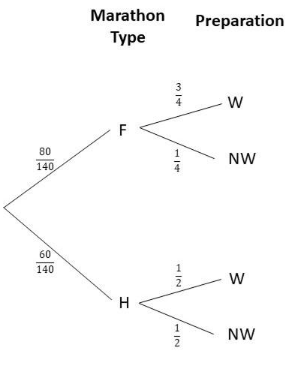b) What is the probability of randomly picking a marathon participant who warms up before running a full-marathon?

c) What is the probability of randomly picking a marathon participant who does not warm up before running?
80 + 60 = 140
total: 140 runners; 90 warm-up.
The probability that a randomly selected runner warms up is 90/140.

Question 15.
The probability of Cindy waking up after 8 A.M. on a weekend day is p. Assume the events of Cindy waking up after 8 A.M. and by 8 A.M. are mutually exclusive and complementary.
a) If p = 0.3, find the probability that she will wake up after 8 A.M. on two consecutive weekend days.
P(waking up after 8 A.M. two weekend days in a row) = p²
If p=0.3
p² = (0.3)²
p² = 0.09

b) If p = 0.56, find the probability that she will wake up by 8 A.M. on two consecutive weekend days.
P(waking up before 8 A.M two weekend days in a row) = (1 – p)²
If p = 0.56, 1-p = 0.44 and
(1 – p)² = (0.44)² = 0.1936.

Question 16.
In a jar, there are 2 raisin cookies and 3 oat cookies. Steven takes two cookies one after another without replacement.
a) Draw a tree diagram to represent the possible outcomes and the corresponding probabilities.
Since there are two different kinds of muffins, which are bran and pumpkin, then start the tree diagram with 2 branches. Now, at the end of these branches put the possible outcomes: P for pumpkin muffin, and B for bran muffins. Note that there are 2 pumpkin muffins out of the total of 5 muffins, and 3 bran muffins out of the 5 total muffins. So the probability for selecting a pumpkin is $$\frac{2}{5}$$, and the probability of selecting a bran is $$\frac{3}{5}$$.

Next, there are stilt 2 different kinds of muffins, then again, at the end of these branches put the possible outcomes: P for pumpkin muffin, and B for bran muffins. If a pumpkin muffin is obtained in the first pick, then on the second pick, there are now 1 pumpkin muffin and a totat of 4 muffins left since a muffin is already taken, then the probability of choosing a pumpkin is $$\frac{1}{4}$$. Also, there are still 3 bran muffin out of 4 muffins left, thus, the probability of selecting a bran muffin is $$\frac{3}{4}$$.

If a bran muffin is obtained in the first pick, then on the second pick, there are still a pumpkin muffin and a total of 4 muffins left since a muffin is already taken, then the probability of choosing a pumpkin is $$\frac{2}{4}$$. Also, there are only 2 bran muffin left out of 4 muffins, thus, the probability of selecting a bran muffin is $$\frac{2}{4}$$. Therefore, the tree diagram for the said event is as depicted below.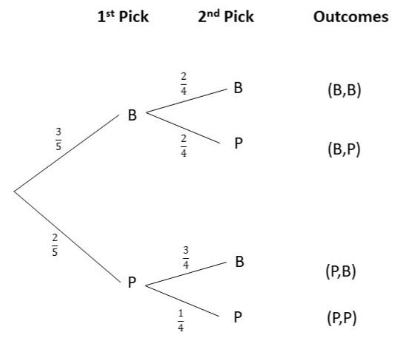b) Find the probability of Steven randomly getting two of the same type of cookie.
The probability of seLecting 2 muffins of a matching type is the probability of obtaining 2 pumpkin muffins, P(P, P), or the probability of choosing 2 bran muffins, P(B, B), then
P(P, P) or P(B, B) = P(P, P) + (B, B)
= P(P) · P(P) +P(B) · P(B)
= $$\frac{2}{5}$$ · $$\frac{1}{4}$$ + $$\frac{3}{5}$$ · $$\frac{2}{4}$$
= $$\frac{2}{20}$$ + $$\frac{6}{20}$$
= $$\frac{8}{20}$$
Therefore, the probability of picking 2 muffins of a matching type is $$\frac{8}{20}$$.

c) Find the probability of Steven randomly getting at least one raisin cookie.
For, the probability of selecting a minimum of one pumpkin muffin, note that the probability of choosing 2 bran muffins, P(B, B), and the probability of picking at least one pumpkin muffin is complementary.
P(B, B) + P(Minimum of One Pumpkin) = 1
P(Minimiun of One Pumpkin) = 1 – P(B, B)
P(Minimum of One Pumpkin) = 1 – P(B) · P(B)
P(Minimum of One Pumpkin) = 1 – $$\frac{3}{5}$$ · $$\frac{2}{4}$$
P(minimum of One Pumpkin) = 1 – $$\frac{6}{20}$$
P(Minimum of One Pumpkin) = $$\frac{7}{10}$$
Therefore, the probabiUty of picking a minimum of one pumpkin is $$\frac{7}{10}$$.

Question 17.
Out of 100 raffle tickets, 4 are marked with a prize. Matthew randomly selects two tickets from the box.
a) Draw a tree diagram to represent the possible outcomes and the corresponding probabilities.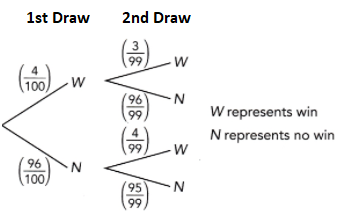b) What is the probability that Matthew does not win any prizes?

c) What is the probability that Matthew gets exactly one of the prizes?

Question 18.
The tree diagram shows the probability of how Shane spends his day gaming or cycling, depending on the weather. The probability of rain is denoted by a. Assume that gaming and cycling are mutually exclusive.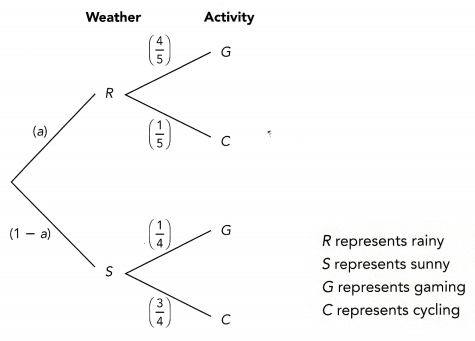a) If a = 0.4, find the probability that he will spend his day gaming.